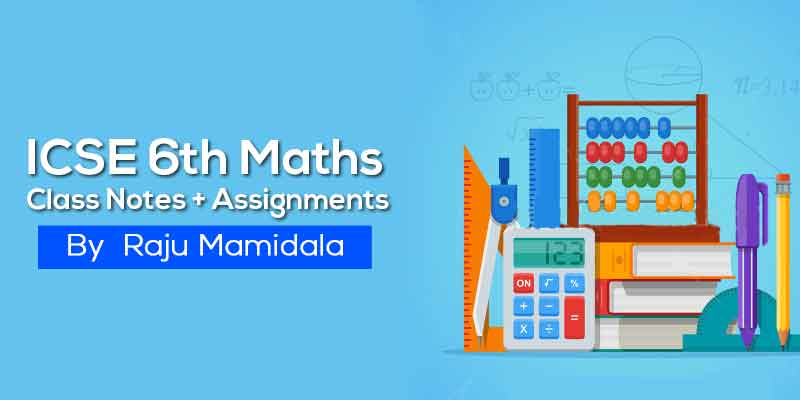## by Raju Mamidala

46 Users Enrolled
Class 6 , Maths
Board: ICSE , Language: English

Mode- Online
Contains- 30 PDFS
Access Duration- Till 30 Apr 2019

## WHAT WILL I LEARN?

We aim to prepare Class 6 ICSE students with a complete study material so that the students can score better in the school exam.

Simple and easy to understand.

Understand the level of IIT Foundation concepts by these quick revision notes.

Student can test themself with assignments which are provided here.

## Course Description

ICSE Class 6 is an important stage where students need to concentrate on this subject as it is considered to be the groundwork of higher classes. To become clear with your aspiration and knowledge, you need to begin your preparation from this level. so here you can get complete notes and assignments for preparation along with solutions/Answers.

This study material will help you to better understand the topics and  these notes, students will be able to make quick revisions of topics and score good marks in there exams.

## Curriculum

Decimals

Fractions

Factors and Multiples

• Factors and Multiples - Class Notes
• Factors and Multiples - Work Assignment

Fundamental Concepts of Algebra, Operations on Algebraic Expressions

• Fundamental Concepts of Algebra, Operations on Algebraic Expressions - Class Notes
• Fundamental Concepts of Algebra, Operations on Algebraic Expressions - Work Assignment

Fundamental geometrical concepts of Lines and Angles

• Fundamental geometrical concepts of Lines and Angles - Class Notes
• Fundamental geometrical concepts of Lines and Angles - Work Assignment

Integers

• Integers - Class Notes
• Integers - Work Assignment

Linear symmetry

• Linear symmetry - Class Notes
• Linear symmetry - Work Assignment

Number system

• Number system - Class Notes
• Number system - Work Assignment

Percentage, Profit and Loss, Simple Interest

• Percentage, Profit and Loss, Simple Interest - Class Notes
• Percentage, Profit and Loss, Simple Interest - Work Assignment

Powers and roots

• Powers and roots - Class Notes
• Powers and roots - Work Assignment

Ratio and proportion

• Ratio and proportion - Class Notes
• Ratio and proportion - Work Assignment

Sets and Venn diagram

• Sets and Venn diagram - Class Notes
• Sets and Venn diagram - Work Assignment

Solids, Perimeter and Area of Plane Figures, Volumes and Surface Area of Solids

• Solids, Perimeter and Area of Plane Figures, Volumes and Surface Area of Solids - Class Notes
• Solids, Perimeter and Area of Plane Figures, Volumes and Surface Area of Solids - Work Assignment

Statistics

• Statistics - Class Notes
• Statistics - Work Assignment

Triangles, Circles and Constructions

• Triangles, Circles and Constructions - Class Notes
• Triangles, Circles and Constructions - Work Assignment

## About Raju Mamidala### Raju Mamidala

Mathematics | Class 6th to Competitive exams | 15+ Years Experience | Working in Geethanjali International School in Hyderabad from past 5 years. He Created an Educational Channel for improvement in various aspects for students in IIT Foundation.

#### If you have any doubts about how to get and access this course,watch this video.## 500 299

Includes 18 % GST

Mode- Online

Contains- 30 PDFS

Access Duration- Till 30 Apr 2019